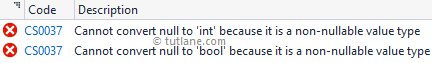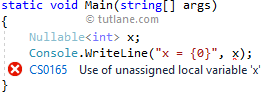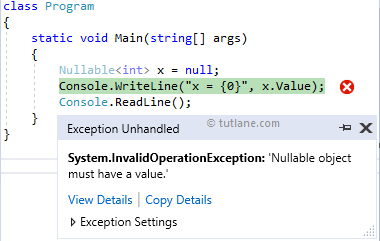# C# Nullable Types

In c#, Nullable Types are useful to assign a null value to the value type variables. In c#, if we try to assign a null value to the value type variables, then we will get a compile-time error.

Following is the simple example of assigning a null value to the value type variables in c#.

using System;

namespace TutlaneExamples
{
class Program
{
static void Main(string[] args)
{
int x = null;
bool y = null;
Console.WriteLine("x = {0}, y = {1}", x, y);
}
}
}

If you observe the above example, we assigned a null value to value type variables (x and y).

When we execute the above code, we will get compile-time errors, as shown below.If you observe the above result, we got a compile-time error because we tried to assign a null value to the value type variables.

To fix this problem, c# has introduced Nullable types to assign a null value to value type variables.

## C# Nullable Syntax

Following is the syntax of declaring variables as nullable types in c#.

Nullable<T> variable_name

or

T? variable_name;

Here, T is an underlying type, and it can be a non-nullable value type, and the Nullable type will represent all the values of an underlying type T and additional null value. The syntax T? is shorthand for Nullable<T> and these two forms are interchangeable.

For example, Nullable<int> can be assigned any integer value from Int32.MinValue (-2147483648) to Int32.MaxValue (2147483647) or a null value. Same way, Nullable<bool> can be assigned a true, false or a null value.

Generally, the nullable types are useful when we perform database operations because sometimes, the fields in database tables will have no value or missing. For example, a field in the database may contain true or false or no value. In that case, we need to use Nullable<bool> or bool? to handle that field value.

## C# Nullable Type Declaration and Assignment

Following is the example of declaring and assigning a value to value type variables using nullable types in c#.

Nullable<int> x = 10;
int? y = 20;
bool? z = null;
Nullable<double> a = null;
int?[] arr = new int?;

If you observe the above example declarations, we used Nullable<T> and T? notations to declare and assign values to nullable types.

Once we declare a nullable type, we must be assigned with some value the same as value type; otherwise, we will get a compile-time exception “Use of unassigned variable” like as shown below.## C# Check Nullable Type Has Value

Before we get or access a value from nullable type, we need to verify whether the instance of nullable type has a value or not by using `HasValue` property otherwise, we will get a runtime exception like as shown below.Following is the example of accessing a value from a nullable type instance by verifying whether it’s having a value or not using `HasValue` property in c#.

using System;

namespace TutlaneExamples
{
class Program
{
static void Main(string[] args)
{
Nullable<int> x = null;
if (x.HasValue)
{
Console.WriteLine("x = {0}", x.Value);
}
else
{
Console.WriteLine("Value is Empty");
}
}
}
}

If you observe the above example, we used the HasValue property to verify whether the nullable type variable (x) is having value or not. The `HasValue` property will return true only when the variable x has value; otherwise, it will return false.

When we execute the above example, we will get the result as shown below.

Value is Empty

We can also compare the nullable type variable with `null` instead of `HasValue` property to check whether the nullable type variable has value or not, as shown in the following example.

int? x = 10;
if (x != null)
{
Console.WriteLine("x = {0}", x.Value);
}
else
{
Console.WriteLine("Value is Empty");
}

## C# Access Nullable Type Value

In c#, we can access nullable type value like `NullableType.Value` like as shown below.

Nullable<int> x = 10;
Console.WriteLine("x = {0}", x.Value);

In the above code, we are able to access the nullable type variable x value like x.Value, but it will throw a runtime exception if the nullable type value is null or not assigned any value like as shown below.So, to access nullable type values, it’s better to use GetValueOrDefault() method. This method will return the default value if the nullable type value is null; otherwise, it will return the actual value.

Following is the example of accessing nullable type value using GetValueOrDefault() method in c#.

using System;

namespace TutlaneExamples
{
class Program
{
static void Main(string[] args)
{
Nullable<int> x = null;
Console.WriteLine("x = {0}", x.GetValueOrDefault());
}
}
}

When we execute the above example, we will get the result as shown below.

x = 0

## C# Assign Nullable Type to Non-Nullable Type

If we want to assign a nullable type value to a non-nullable type, then we need to use a null-coalescing operator `??` to specify the value to be assigned if the nullable type value is null; otherwise, we will get a runtime exception.

Following is the example of assigning nullable type value to a non-nullable type using a null-coalescing operator `??` in c#.

using System;

namespace TutlaneExamples
{
class Program
{
static void Main(string[] args)
{
int? x = null;
// y = x if x is not null, y = 0 if x is null
int y = x ?? 0;
Console.WriteLine("y = {0}", y);
}
}
}

If you observe the above example, we used a null-coalescing operator (`??`) to assign a nullable type variable x value to variable y and specified the value (0) to be assigned to variable y if nullable type variable x value is null.

When you execute the above program, you will get the result as shown below.

y = 0

## C# Nullable Types Characteristics

Following are the important characteristics of the nullable type in c#.

• Nullable types are useful to assign a null value to the value type variables.
• Nullable types can declare like either` Nullable<T>` or `T?`. The syntax `T?` is shorthand for Nullable<T> and these two are interchangeable.
• We can assign a value to nullable type like `int? i = 10` or `Nullable<int> i = 10` or `int? i = null`.
• By using a nullable type `HasValue` and Value properties, we can verify and retrieve the variable value like `if(i.HasValue) j = i.Value;`.
• The `HasValue` property will return true if the variable contains a value or false if it is null.The Value property will return value if the variable contains a value; otherwise, it will throw an InvalidOperationException exception.
• We can use GetValueOrDefault() method to get the value from nullable type. It will return the value of nullable type if it contains a value or if it is null, then it will return the default value.
• To assign nullable type to non-nullable type, then we need to use the null-coalescing operator `??`.
• Nested nullable types are not allowed like `Nullable<Nullable<int>> x.`